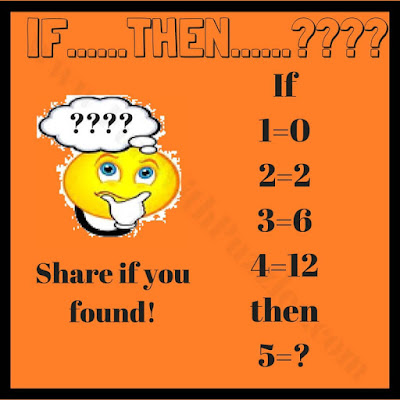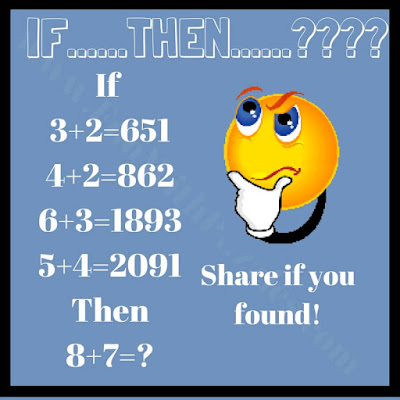Challenge middle school students with engaging logic puzzles and math questions. Enhance critical thinking and problem-solving skills with these captivating activities.

There are many different kinds of logical puzzles. There are logical puzzles in which one story runs and at the end, one has to logically deduct the answer. There are also logical puzzles in which a sequence of numbers is given and one has to tell logically which number will come next. Also, there are many favorite car parking logical problems. Do try these puzzles if you like to solve logical puzzles. There is a very interesting logical puzzle type in which statements are given and then one has to find the logic in these equations and deduct the answer.

In these puzzles, there are some equations given which if read logically are correct and follow a certain mathematical pattern. One has to deduct the logical pattern in these equations and then solve the last equations.

We are posting these puzzles in order from easy to tough. However difficulty level of the puzzles varies from individual to individual. Let us see how many of these puzzles you can solve quickly? Do post your answers in the comment box of this post. Answers to these Brain Maths Logic Puzzles are given in this post at the end.1. Can you solve it?2. Can you find the missing number?3. Can you find this missing number?4. If you can solve it, you are genius5. Can you solve this tough logical picture puzzle?

## Answers to Logic Puzzles or Math Questions

These kinds of Maths Logic Brain Puzzles test your both Mathematical skills as well as Logical Reasoning. Below is a list of similar puzzles which you should try out to increase your brainpower.

## List of Maths and Logical Puzzles

1) Maths Logic Puzzles Brain Teasers with Answers: In these Maths Logic Puzzles, some Logical Equations are given that follows certain Mathematical pattern. Your challenge is to find this pattern and then solve the last equation.

2) River Crossing Brain Challenges with Answers: In these logical river crossing brain challenges, your task is to use your logical reasoning to help some people to cross the bridge.

3) Most Tricky Questions in Pictures: These are very tricky logical questions that are presented in the pictures.

4) Tricky Maths Brain Challenges with answers: At last these Circle Maths Hard Brian Challenges in which your challenge is to find the missing number around the Circle which will replace the question mark.